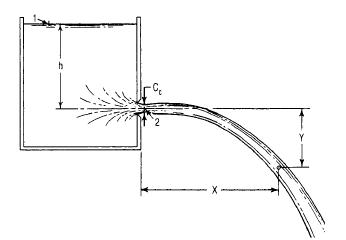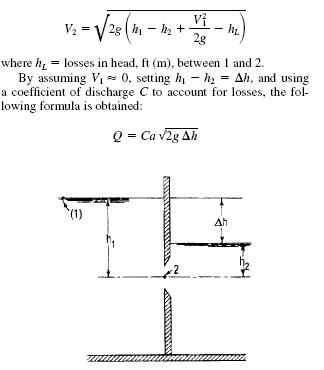Search

# Flow Through Orifices.

Posted in Hydraulics |Email This Post |

Orifice Discharge into Free Air

An orifice is an opening with a closed perimeter through which water flows. Orifices may have any shape, although they are usually round, square, or rectangular.

Discharge through a sharp-edged orifice may be calculated from:

Q = Ca?2gh

where
Q= discharge, ft3/s (m3/s)

C =coefficient of discharge

a =area of orifice, ft2 (m2)

g =acceleration due to gravity, ft/s2 (m/s2)

h =head on horizontal center line of orifice, ft (m)

The coefficient of discharge C is the product of the coef- ficient of velocity Cv and the coefficient of contraction Cc. The coefficient of velocity is the ratio obtained by dividing the actual velocity at the vena contracta (contraction of the jet discharged) by the theoretical velocity. The theoretical velocity may be calculated by writing Bernoulli’s equation for points 1 and 2.ThusV2= ?2gh

The coefficient of contraction Cc is the ratio of the smallest area of the jet, the vena contracta, to the area of the orifice.

Submerged Orifices

Flow through a submerged orifice may be computed by applying Bernoulli’s equation to points 1 and 2 in figure belowValues of C for submerged orifices do not differ greatly from those for nonsubmerged orifices.